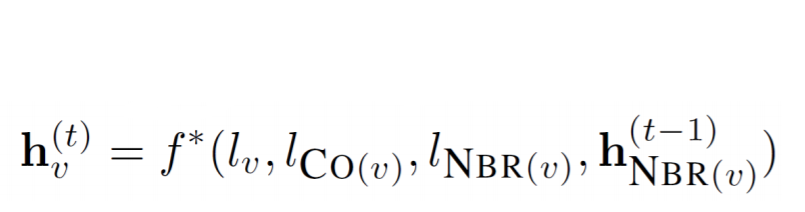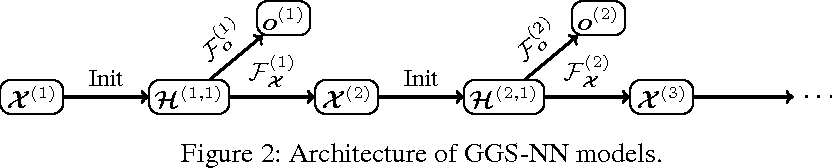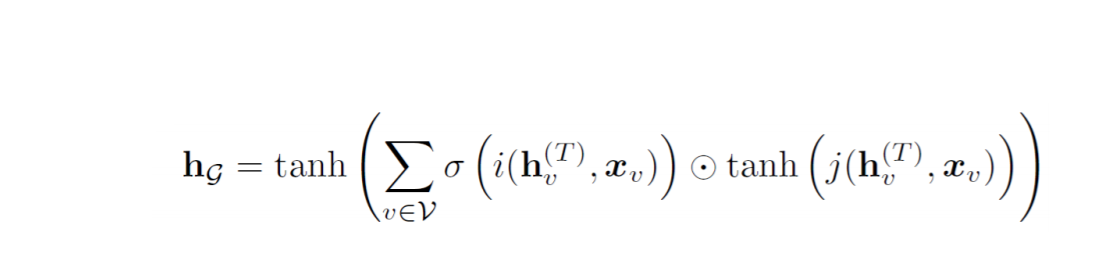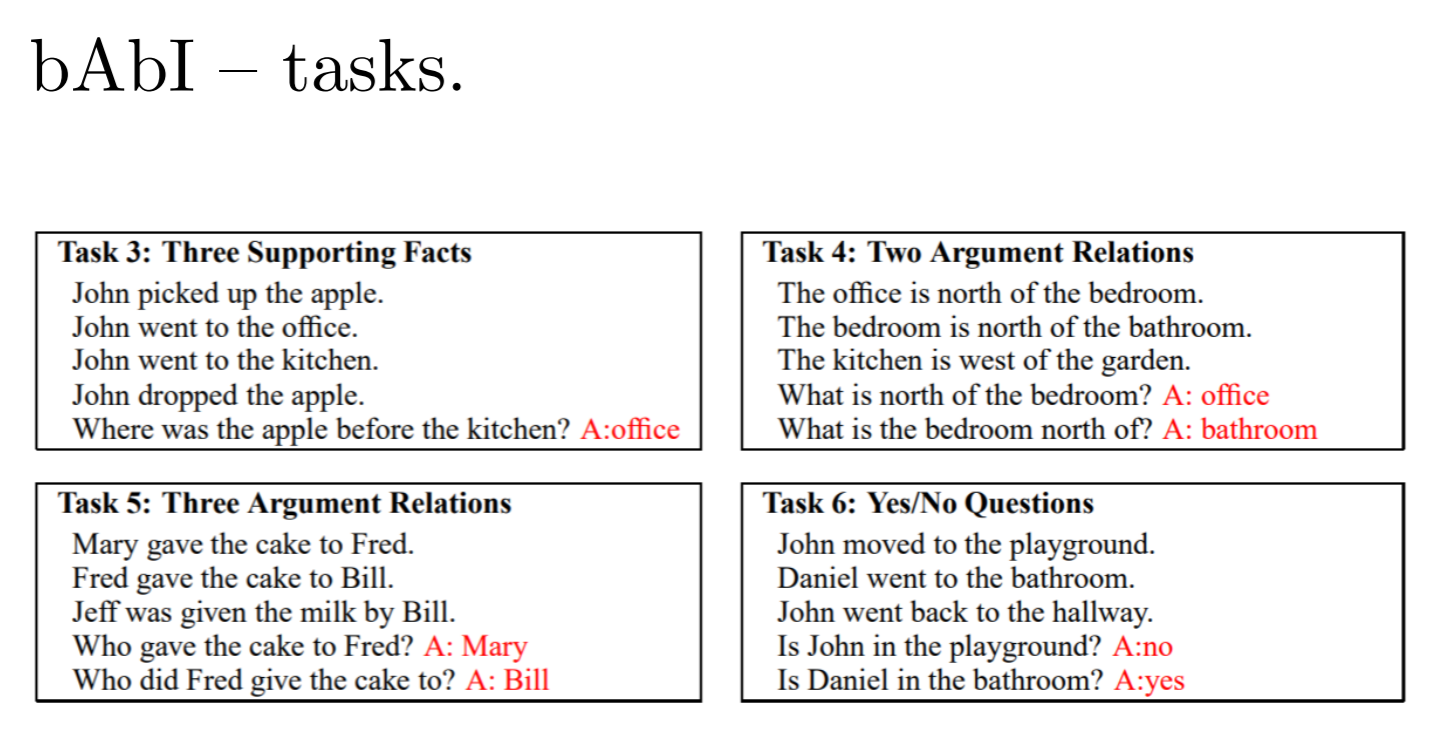×

Search anything:

# Gated Graph Sequence Neural Networks (GGSNN)

#### Machine Learning (ML)Get this book -> Problems on Array: For Interviews and Competitive Programming

Gated Graph Sequence Neural Networks (GGSNN) is a modification to Gated Graph Neural Networks which three major changes involving backpropagation, unrolling recurrence and the propagation model. We have explored the idea in depth.

We start with the idea of Graph Neural Network followed by Gated Graph Neural Network and then, Gated Graph Sequence Neural Networks.

Graph Neural Networks are great at some of the most complicated databases like social networking site data or logistic services and even in natural language semantics.

Graph structure data are highly prevalent, and when we want to learn the features over the graph data, we use GNNs. The existing work as we have seen in the previous article on the Introduction to Graph Neural Networks deals with two things. First, the global outputs and second, the individual node-wise predictions.

### Changes

But what if we want to work with sequential data? We do not have any system to learn the features from sequential graph data. This can be achieved by making changes to the existing methodology of the GNNs.

Let us quickly revise the methodology of GNNs. There are two big steps involved.
First, the node computations, for example, the recurrent nodes.
Second, the forward propagation step, i.e. the data propagation step (aka message passing).

### Node computations

So, the first thing we need to change is the node computations. We want sequential outputs on our nodes. This will require to encode or even approximate the sequence so far. This will lead to a clear sequence of computations and would help us in achieving the computations from all the nodes.

#### Propagation

The second thing was to change the overall propagation step to take into consideration the inputs from previous node sequences. This was done by replacing the feed-forward propagation by Gated Recurrent Units (GRUs).

Earlier the propagation step for the GNNs was to collect all the neighboring node values and have a summation over it and pass an activation function through that value. The changed propagation step consists of an implementation of the Almeida-Pineda Algorithm.

Below is the simple structure equation for the same.This makes it easier to run the propagation to convergence. And thus, we can compute the gradients on the converged solution.

## GGNN to GGSNN

This was named Gated Graph Neural Networks. To convert these to Gated Graph Sequence Neural Networks, we need to do the following with the core ideas:

• Replace the propagation model with GRU (Already in GGNN)
• Unroll recurrence for T time-steps. Rather than unrolling till convergence.
• Use backpropagation-through-time to compute gradients.

Now as we know the basic motivation behind the Gated Graph Sequence Neural Networks (GGSNN), let us quickly introduce you to the architecture of the same. This will make it easier for you to understand the working.
Look at the below architecture.Here we need to define two things for you, first the node annotations (x) and second is the F(x) function. Which defines the shared propagation models.

• Node annotations: The GGSNN does allow for initializing the nodes with additional inputs (x). This allows us to supply the previous node inputs as well as any other initialization required. X denotes if the node is special. Here in the above equation X means the matrix of all the node annotations.

• The other thing is the H. Those are nothing but the node representations.

Here we rely on the function f* for the convergence. But once we use the GGSNN, we simply check on the unrolling index for the termination of the algorithm.
It is important to note that, once we expand the f*, we find that the base function is a simple linear transformation or a feed-forward network. So, it still keeps the base functionality of a GNN into the architecture.

This covers the sequential computations for the network. Now coming to the final step, which is the output of the network.

The node-level outputs here are calculated by simply passing the values through a function g(). Here, g is a differential function that maps the node embeddings as well as the labels to the output.

#### Global Output

For the global outputs, we may use the following equation.Now with a clear and thorough understanding of each step let us dive into numbers. Let us see how it performed compared to other state-of-the-art algorithms. For making the tests more accurate the researchers formed a special tasks list. Also known as the bAbI task list.

#### Experimental results

The experiments consisted of 20 such tasks and the accuracies were measured for every such task.

bAbI Task 4 97.3±1.9 (250) 97.4±2.0 (250) 100.0±0.0 (50)
bAbI Task 15 48.6±1.9 (950) 50.3±1.3 (950) 100.0±0.0 (50)
bAbI Task 16 33.0±1.9 (950) 37.5±0.9 (950) 100.0±0.0 (50)
bAbI Task 18 88.9±0.9 (950) 88.9±0.8 (950) 100.0±0.0 (50)

These are the tasks and the accuracies with the number of steps required for it to reach those accuracies. These tasks dealt with the deduction of a certain data story input; an example can be seen in the below image.Do note, these are not cherry-picked results, the GGSNN also beats the other tasks but these were the closets the networks could come to the perfect accuracy of GGSNN.
Other tasks involved were, learning graph algorithms.
The accuracies on that were as follows:

bAbI Task 19 24.7±2.7 (950) 28.2±1.3 (950) 71.1±14.7 (50) 92.5±5.9 (100) 99.0±1.1 (250)
Shortest Path 9.7±1.7 (950) 10.5±1.2 (950) 100.0± 0.0 (50)
Eulerian Circuit 0.3±0.2 (950) 0.1±0.2 (950) 100.0± 0.0 (50)

So, we do see the change in accuracy once we shift to GGSNN. The algorithm is a definite improvement over the standard GNNs, and these would help improve the overall graph-structure data analysis and feature learning tasks.

With this article at OpenGenus, you must have the complete idea of Gated Graph Sequence Neural Networks (GGSNN). Enjoy.